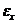# The strains for an element of material in plane strain (see figure) are as follows: ε x = 480 ×10 -6 . ε y = 140 × l0 -6 , and γ x y = —350 x 10”. Determine the principals strains and maximum shear strains, and show these strains on sketches of properly oriented elements.### Mechanics of Materials (MindTap Co...

9th Edition
Barry J. Goodno + 1 other
Publisher: Cengage Learning
ISBN: 9781337093347
Chapter 7, Problem 7.7.11P
Textbook Problem
116 views

## The strains for an element of material in plane strain (see figure) are as follows: ε x = 480 ×10-6. ε y = 140 × l0-6, and γ x y = —350 x 10”. Determine the principals strains and maximum shear strains, and show these strains on sketches of properly oriented elements.To determine

The principal strains and maximum shear strain.

### Explanation of Solution

Given:

Normal strain along x-direction is 480×106, and normal strain along y direction is 140×106and the shear strain along x-y plane is 350×106.

Write the expression for the principal strains.

ε1,2=εx+εy2+(εxεy2)2+(γxy2)2        …… (I)

Here, the maximum principal strain is ε1, the minimum principal strain is ε2.

The normal strain along x direction is εx, the normal strain along the y direction is εyand shear strain along x-y plane is γxy.

Write the expression for maximum shear strain.

γmax=ε1ε2     …… (II)

Here, the maximum shear strain is γmax.

Write the expression for principal strain angle.

tan2θp1=γxyεxεy        …… (III)

Here, principal strain angle is θp1.

Write expression for orientation of first shear strain angle.

θs1=θp145°        …… (IV)

Here, first shear angle is θs1.

Write expression for orientation of second shear strain angle.

θs2=θs1+90°     …… (V)

Here, second shear angle is θs2.

Calculation:

Substitute 480×106for εx, 140×106for εyand 350×106for γxyin Equation (I).

ε1,2=480×106+140×1062±(480×106140×1062)2+(350×1062)2=310×106±2.89×108+3

### Still sussing out bartleby?

Check out a sample textbook solution.

See a sample solution

#### The Solution to Your Study Problems

Bartleby provides explanations to thousands of textbook problems written by our experts, many with advanced degrees!

Get Started

Find more solutions based on key concepts
What are cryptography and cryptanalysis?

Principles of Information Security (MindTap Course List)

What are the basic characteristics of a NoSQL database?

Database Systems: Design, Implementation, & Management

Explain why database design is important.

Database Systems: Design, Implementation, & Management

Obtain information on the standard sign typefaces used for highway signs in theUnited States. The Federal Highw...

Engineering Fundamentals: An Introduction to Engineering (MindTap Course List)

Compare and contrast at least three websites or apps that can generate QR codes. Which app would you use to gen...

Enhanced Discovering Computers 2017 (Shelly Cashman Series) (MindTap Course List)

If your motherboard supports ECC DDR3 memory, can you substitute non-ECC DDR3 memory?

A+ Guide to Hardware (Standalone Book) (MindTap Course List)